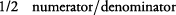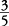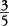## What Are Fractions?

A fraction, or fractional number, is used to represent a part of a whole. Fractions consist of two numbers: a numerator (which is above the line) and a denominator (which is below the line).orThe denominator tells you the number of equal parts into which something is divided. The numerator tells you how many of these equal parts are being considered. Thus, if the fraction isof a pie, the denominator 5 tells you that the pie has been divided into 5 equal parts, of which 3 (numerator) are in the fraction. Sometimes, it helps to think of the dividing line (the middle of a fraction) as meaning “out of.” In other words,also means 3 out of 5 equal pieces from the whole pie.